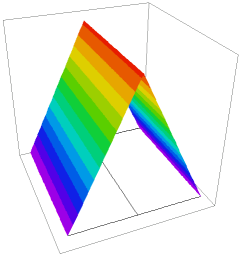﻿ Sample Problems > Usage > Contact_Boundaries > contact_resistance_heating

contact_resistance_heating

Navigation:  Sample Problems > Usage > Contact_Boundaries >

contact_resistance_heating{  CONTACT_RESISTANCE_HEATING.PDE

Contact resistance is modeled using the keywords JUMP and CONTACT.

JUMP represents the "jump" in the value of a variable across an interface

(outer value minus inner value, as seen from each cell),

and is meaningful only in boundary condition statements.

CONTACT is a special form of NATURAL, which requests that the boundary

should support a discontinuous value of the variable.

The model is one of "contact resistance", where the outward current across an

interface is given by

R*I = -Jump(V) [=(Vinner-Vouter)],

and R is the contact resistance.

Since CONTACT, like NATURAL, represents the outward normal component

of the argument of the divergence operator,  the contact resistance condition

for this problem is represented as

CONTACT(V) = JUMP(Temp)/R

In this problem, we have two variables, voltage and temperature.

There is an electrical contact resistance of 2 units at the interface between

two halves, causing a jump in the voltage across the interface.

The current through the contact is a source of heat in the temperature equation,

of value P = R*I^2 = Jump(V)^2/R

 }   title "contact resistance heating"   variables    V    Temp   definitions    Kt     { thermal conductivity }    Heat = 0    Rc = 2   { Electrical contact resistance }    rho = 1   { bulk resistivity }    sigma = 1/rho { bulk conductivity, I=sigma*grad(V) }    temp0=0    size = 3    V1 = 1    totR = size*rho+Rc    cur = V1/totR    jdrop = cur*Rc initial values     Temp = temp0   equations    V:       div(sigma*grad(V))  = 0    Temp:    div(Kt*grad(Temp)) + Heat =0   boundariesRegion "R1"

Kt=5

start 'box' (0,0)

natural(V)=0 natural(temp)=0 line to (size,0)

value(V)=V1 value(temp)=0   line to (size,size)

natural(V)=0 natural(temp)=0 line to (0,size)

value(V)=0   value(temp)=0   line to close

Region "R2"

Kt=1

start (0,0)

line to (size/2,0)

contact(V) = (1/rc)*JUMP(V) { resistance jump }

natural(temp) = JUMP(V)^2/Rc   { heat generation }

line to(size/2,size)

natural(V)=0

natural(Temp)=0

line to (0,size) to close

Feature 'interface' start (size/2,0) line to (size/2,size)

monitors

contour(Temp)

plots

grid(x,y)

contour(V) painted

contour(Temp) painted

surface(Temp)

contour(kt*dx(temp)) painted

contour(kt*dx(temp)) painted

elevation(V) from(0,1.5) to (3,1.5)

elevation(temp) from(0,1.5) to (3,1.5)

elevation(dx(v)) from(0,1.5) to (3,1.5)

elevation(kt*dx(temp)) from(0,1.5) to (3,1.5)

summary

report(sintegral(V,'interface','R1')/size)   ! find average interface voltage in region 1

report(sintegral(V,'interface','R2')/size)   ! find average interface voltage in region 2

report(sintegral(jump(V)^2/rc,'interface')) as "contact source"